Journal of Electrical and Electronic Engineering
Volume 4, Issue 2, April 2016, Pages: 24-30

A New Method for Ground Moving Targets Tracking Using Radar Based on Compressed Sensing

Wang Xue-Jun

School of Electronic Information Engineering, Beihang University, Beijing, China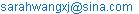Wang Xue-Jun. A New Method for Ground Moving Targets Tracking Using Radar Based on Compressed Sensing. Journal of Electrical and Electronic Engineering. Vol. 4, No. 2, 2016, pp. 24-30. doi: 10.11648/j.jeee.20160402.14

Received: January 26, 2016; Accepted: March 1, 2016; Published: April 7, 2016

Abstract: In this paper, we propose a Compressed Sensing (CS) based method under the unknown sparse degree to track ground moving targets using Pulse-Doppler (PD) radar. We use the sparsity of delay-Doppler plane in the process of disposing PD radar echo to set up a sparse signal model in each pulse interval. At the state prediction stage, we can get the predicted values of target states by dynamic equations, with which we can build a delay-Doppler grid that is used to form orthogonal dictionary. At the state update stage, we can get the target state estimation through reconstruction algorithm, so as to realize precise tracking of targets. The problem of target tracking by PD radar will be transformed into the reconstruction of the sparse signal, which is accomplished by getting the location of targets in the grid, as a result of achieving ground target tracking based on Orthogonal Matching Pursuit (OMP) . Then, aiming at the sparsity problem in the method of target tracking based on Orthogonal Matching Pursuit, we propose a new target tracking method based on Sparsity Adaptive Matching Pursuit (SAMP) algorithm . Numerical simulations show that our tracking method can not only provide the equivalent computational time, but also get better tracking performance than the KF-based tracking.

Keywords: Compressed Sensing (CS), Pulse-Doppler (PD) Radar, Target Tracking, Orthogonal Matching Pursuit (OMP), Sparsity Adaptive Matching Pursuit (SAMP) Algorithm

1. Introduction

Target tracking by radar is the process that people use all kinds of observation and computing methods to realize the procedure of target states modeling and tracking using radar. This technology is widely used in the military and civil fields, such as airborne early warning, multi-target attack, ballistic missile defense, Marine monitoring, battlefield surveillance in military field and traffic control system, intelligent traffic control system in civil field.

In target tracking of radar, the environment generally includes air targets, ground targets and sea targets. Among them, the air and sea targets are relatively easy to track due to the little effect of environment and their research is also gradually mature from the perspective of international literature. However, the ground target tracking has some certain practical difficulties, such as the limitations of terrain shade, minimum detectable rate, the seriousness of ground clutter, the restriction of road network, the density and flexible of targets, etc.

CS is provided to alleviate the pressure of huge amount of information demand caused by the signal sampling, transmission and storage . Compared with the traditional Nyquist sampling theorem, the difference of CS is that we can use a observation matrix reversing the basic shift matrix to make the transformation of high-dimensional signals cast onto a low-dimensional space, and reconstruct the original signal with a high probability by solving a nonlinear problem . AS the CS theory can reduce the cost of sampling and computing, it has important influences and practical significance on many disciplines and fields. In recent years, some experts and scholars applied the CS theory to the radar signal processing and obtained many important achievements    .

In this paper, we prove that the targets are sparse in delay-Doppler plane through the establishment of delay-Doppler grid and achieve accurate reconstruction of signals after realizing the sparse representation of echo signal by setting up orthogonal dictionary. In addition, we use the particularity of the grid to search for location of the grid corresponding to the largest coefficient while rebuild signals. Finally, we get the accurate approximation of target measurements.

We mainly discuss the ground target tracking method based on CS using PD radar, analyze the features of receiving echo  and apply the CS theory to the tracking procedure , which can reduce the computational time and improve the tracking accuracy. Therefore, the research of this dissertation has very vital significance.

2. Tracking Model

2.1. Target Dynamic Model

Suppose that there are m targets moving in a straight line in our interesting area and each target’s motion state can be showed by its position and velocity, so we can get the mth target’s dynamics at the kth pulse interval as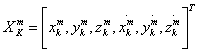However, the PD radar track the targets by the delay and Doppler instead of position and velocity. In the kth pulse interval, the delay and Doppler of mth target could be described as: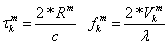where Rm denotes the distance between the mth target and radar and Vm denotes the radial velocity of mth target. If the initial position of radar is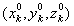, then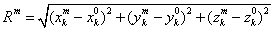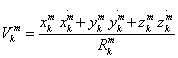Hence, we can form a state dynamic equation in kth pulse interval, as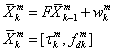F denotes state transform matrix,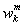denotes the state model error, and T denotes the state sampling interval, which is equal to the pulse repetition interval (PRI).

2.2. PD Measurement Model

We use the linear frequency modulation (LFM) pulse signal as the transmitting signal, and suppose its pulse width as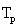, carrier frequency as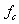, bandwidth as B, so the emission signal can be represented as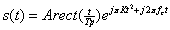(1)

Where K=B/Tp denotes the modulation slope. Suppose there are m targets moving in our interesting area, then the echo signal in the kth pulse interval can be expressed as: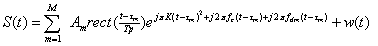(2)

We can get the sampling signal after sampling of S(t) with sampling interval ts=1/fs=1/2B, as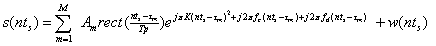(3)

Where n=1, 2, 3...N denotes the number of sampling, and let Am=1. If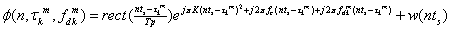(4)

Then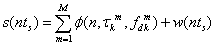(5)

Where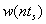denotes Gaussian noise and obeys the normal distribution of N(0,Q),where Q is noise covariance.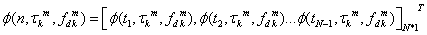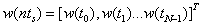2.3. Sparse Measurement Model

In order to apply the sparsity of delay-Doppler to target tracking procedure, we study an equivalent sparse representation method of echo signal model in PD radar. In the kth pulse interval, we discrete the delay-Doppler plane into N1×N2 grids, and if the number of targets M<<N1×N2, then the targets are sparse in delay-Doppler plane . After converting the measurement model of echo signal into the corresponding sparse measurement model, the position of nonzero elements in the sparse vector correspond to the position of targets in grid. The size of resolution for delay and Doppler in grid is determined by the signal bandwidth and pulse duration, as 1/2B and 1/T . The size of resolution limits the size of grid, so the grid partition can’t be infinite. Under the same target scene and tracking conditions, we generally use the same grid resolution . As is show in Figure 1, It’s the grid of N1×N2.

The value that is corresponding to the grid point is show in Figure 2.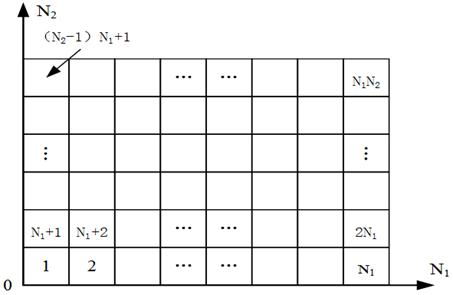Figure 1. Delay-Doppler grid.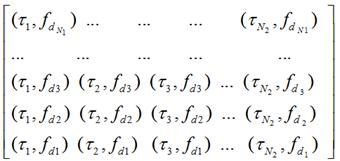Figure 2. Values grid points.

Then we can get the sparse expression of echo signal as: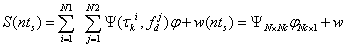(6)

Of which Nc=N1×N2 denotes the sum of grids,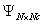denotes the sparse dictionary and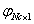denotes sparse coefficient matrix.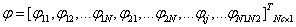2.4. Observation of Sparse Signal

According to the principle of CS, sparse signal must remain the geometric properties under the influence of observation matrix, so if we want to get a perfect reconstruction, we must make sure that the observation matrix won’t let the two different k-sparse signal map into the same sample collection, and this requests the matrix consisted of m column vectors from observation matrix is singular. Some relevant scholars proved that the observation of signals won’t destroy the geometry nature of signals when the observation matrix and sparse matrix are irrelevant . The random Gaussian matrix has a special nature: For a M×N random Gaussian matrix, when meets M>=CKlogN/K (K denotes the sparsity of signal), it can guarantee high precision to restore the original signal . So we choose the random Gaussian matrix as the observation matrix and we can get the observation vector containing original signal information after projecting the sparse signal to measurement matrix.

3. Tacking Filter

Typical target tracking procedure is generally consisted of two stages, namely the predict stage and update stage. In the prediction stage, The predicted state at kth pulse interval can be obtained by the target’s estimated state at k-1th pulse interval as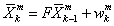.

Then, in the update stage, we can get the target’s estimation state at the kth pulse interval using new measurement values to modify the predicted state. And the OMP algorithm can be used to reconstruct the signal in the update stage so that we can get the best sparse approximation of objective measurement values.

3.1. OMP-Based Ground Target Tracking Using PD Radar

OMP algorithm belongs to greed iterative algorithm avoiding the optimization problem of solving minimum L0 norm. It uses the prior information of signal sparsity to choose the most matching column vector from measurement matrix to construct the sparse approximation of original signal. In the procedure of target tracking by PD radar, we firstly make the sparsity decomposition of receipt signal and then we will get the sparse vector containing target’s measurement information. Here is the process of OMP-based ground target tracking using PD radar.

1) Calculate the target’s prediction state in the kth pulse interval by the target dynamic equation and set up the delay-Doppler grids by the target’s prediction state;

2) Build the sparse dictionary according to the grid values, and make sparse decomposition of original echo signal ;

3) Initialize the allowance as original echo signal and compute the correlation coefficient of allowance and sparse dictionary, which is to make inner-products of allowance and each column in sparse dictionary;

4) Save the column corresponding to the largest absolute value to the index set and put this column in a support set;

5) Use the least square method to make sparse approximation of signal;

6) Update the allowance;

7) Continue the iteration until the allowance is less than a certain minimum value;

8) The column serial number in index set corresponding to the location of grid is the target’s location.

3.2. SAMP-Based Target Tracking Using PD Radar

In the reconstruction process, the OMP algorithm is relatively simple and fast. But the OMP algorithm itself needs to attain the accurate reconstruction under the condition of known sparse degree . In fact, the sparse degree of signal is usually unknown, for that the number of target in delay-Doppler plane is unknown before the sparsity estimation. Aiming at this problem, we put forward a method of SAMP-based target tracking using PD radar that can tracking target in the condition of unknown sparsity. The tracking procedure is as follows.

1) Calculate the target’s prediction state in the kth pulse interval by the target dynamic equation and set up the delay-Doppler grids by the target’s prediction state;

2) Build the sparse dictionary according to the grid values, and make sparse decomposition of original echo signal , the sparsity of signal is unknown;

3) Initialize the allowance as original echo signal and compute the correlation coefficient of allowance and sparse dictionary, which is to make inner-products of allowance and each column in sparse dictionary;

4) Save the columns corresponding to the first L absolute values to the candidate set and use the least square method to make original sparse approximation of signal;

5) Save the columns of candidate corresponding to the first L absolute values to support set and use the least square method to make final sparse approximation of signal.

6) Update the allowance, if it’s bigger than original value, then change to the next stage and increase L;

7) Otherwise, continue the iteration until the allowance is less than a certain minimum value;

8) The column serial number in index set corresponding to the location of grid is the target’s location.

4. Numerical Simulations

In this section, we will show the performance of CS-based target tracking using PD radar through the numerical simulations. We will describe and discuss the simulation results. As the target’s prediction state provides a good approximation of estimation state in each pulse interval, so we can only choose a part of delay-Doppler plane which contains all of the delay-Doppler values in our interesting area to set up the grid according to the target’s prediction state in kth pulse interval .

Our coordinate system is based on inertial coordinate system, and the ground projection point of radar’s original position is the origin of coordinates with the north direction for OX axis, the west direction for OY axis, and the line which is vertical to OX-OY plane for the OZ axis. The PD radar at an altitude of 1km is moving with a speed of 30m/s in a straight line. It’s original position is as (0, 0, 1000) m, carrier frequency is as fc=1Ghz, bandwidth is as B=100MHz, pulse width is as Tp=0.05us, pulse repetition interval is as PRI=10ms. There is one target moving in our interesting area, and it’s original position is (3000,150,0)m, the component speed of OX axis is 10m/s and 15m/s is for OY axis. Assume that the target is making uniform linear motion and the noise is Gaussian noise with SNR=10db. Keep the delay resolution of 3.33ns (corresponding to the range resolution of 0.5m), and keep Doppler resolution of 6.7Hz (the speed resolution of 1m/s).

4.1. Numerical Simulations of OMP-Based Ground Target Tracking Using PD Radar

Firstly, we use OMP-based method to track the round target and compare its performance with KF filter. The results of numerical simulations are as follows.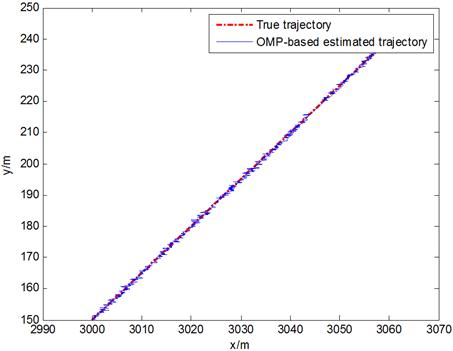Figure 3. Motion curve.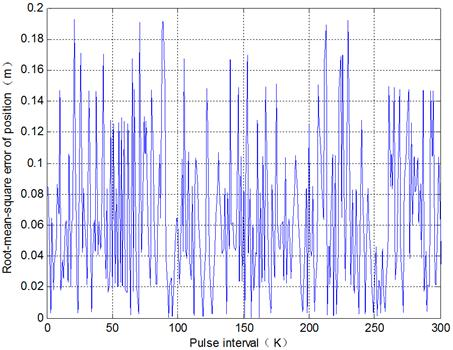Figure 4. Root-mean-square error of position.

The OMP-based approach took 3.24s to estimate the target state of 300 pulse intervals on average. We compare this tracking result with KF filter of which the simulation results are as follows.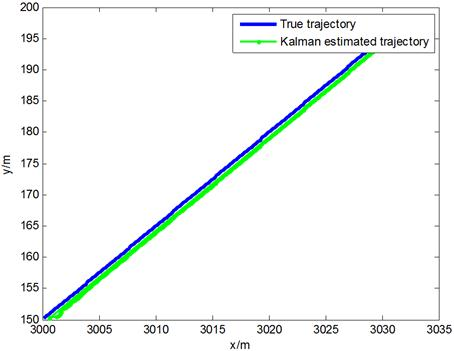Figure 5. Motion curve.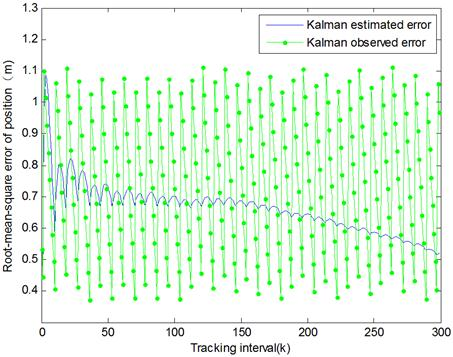Figure 6. Root-mean-square error of position.

The KF filter method took 2.84s to estimate the target state of 300 pulse intervals on average. And their contrast is as follows.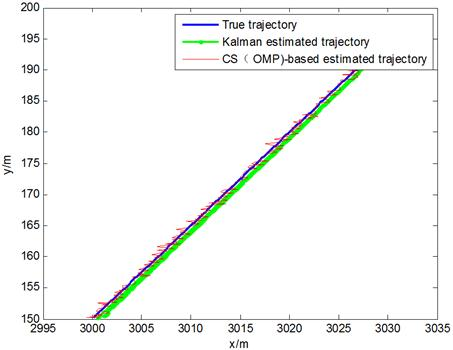Figure 7. Motion curve.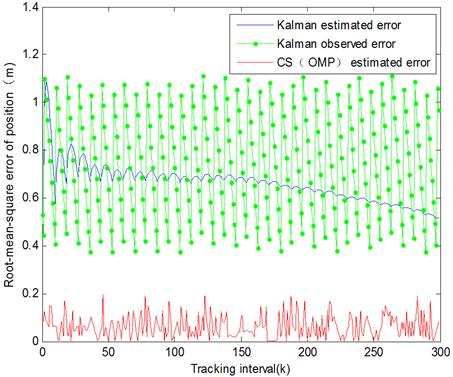Figure 8. Root-mean-square error of position.

From Figure 6 and Figure 7, we found that the OMP tracking approach could provide better precision with corresponding time compared with the traditional KF filter.

4.2. Numerical Simulations of SAMP-Based Ground Target Tracking Using PD Radar

In view of the fact that the OMP-based method needs the known sparsity of signal in advance, we develop a new method of SAMP-based ground target tracking method using PD radar. The data of simulation is the same as OMP-based method.

In SAMP-based method, the option of L is an important point. According to the literature , the range of L is as 1LK, where k is the sparsity of signal. As this paper is about single target tracking, so we let L=1.

We use SAMP-based to track ground target and compare its performance with OMP-based method. The simulation results are as follows.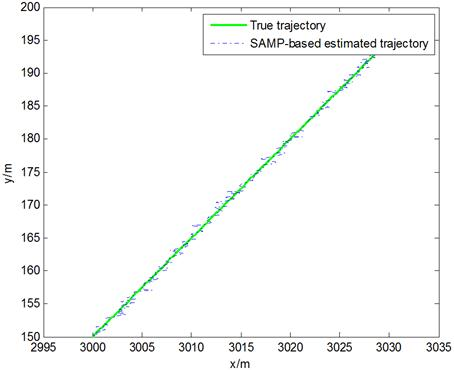Figure 9. Motion curve.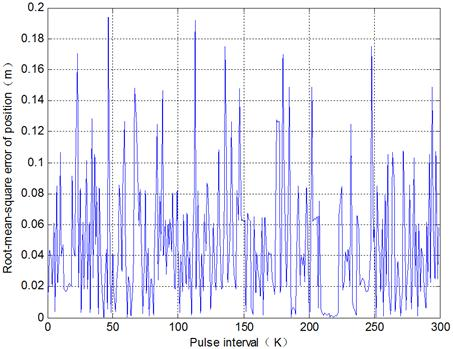Figure 10. Root-mean-square error of position.

The SAMP-based method costs 3.85s to estimate the target state of 300 pulse intervals on average. We compare this tracking result with OMP-based method and the simulation results are as follows.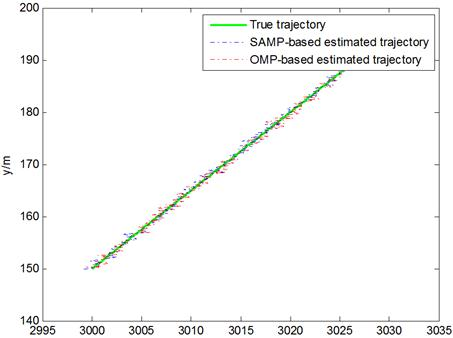Figure 11. Motion curve.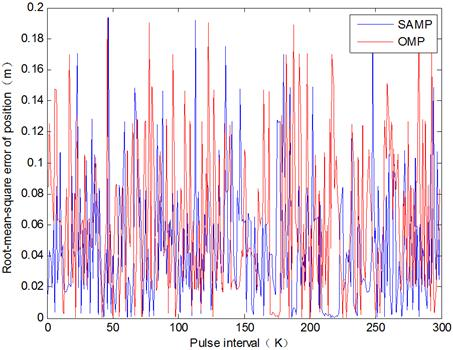Figure 12. Root-mean-square error of position.

From Figure 10 and Figure 11, we can see that SAMP-based method not only solve the problem of unknown sparsity, but also provide the considerable tracking precision.

In conclusion, compared with the traditional KF filter, our CS-based ground target tracking method using PD radar can provide better precision while costs the quite computational time.

5. Conclusion

In this paper, we proposed a Compressed Sensing (CS) based method under the unknown sparse degree to track ground moving targets using Pulse-Doppler (PD) radar. Basing on the analysis of PD radar echo, we transformed the target tracking problem into the reconstruction of sparse signal in CS theory through setting up the orthogonal dictionary by dispersing the delay-Doppler plane.

The simulation results showed that our OMP-based ground target tracking method could provide better precision than traditional KF filter under the considerable computational time. In addition, the SAMP-based ground target tracking method could not only resolve the problem of target tracking with unknown sparsity, but also provide a better tracking performance than KF filter. However, there were some shortcomings in this paper, such as without considering the effect of ground clutter . In the future, we will extend the CS-based ground target tracking method to multi-targets tracking and let the targets scene conform to the realistic conditions.

References

1. Joel A. Tropp, Anna C. Gilbert. Signal Recovery From Random Measurements Via Orthogonal Matching Pursuit[J]. IEEE Transactions on Information Theory, 2007. Vol.53, No.12.4655-4666.
2. Thong T.Do, Lu Gan, et al. Sparsity Adaptive Matching Pursuit Algorithm for Practical Compressed Sensing[C]. Asilomar Conference on Signals, Systems and Computers, Pacific Grove, California, 2008, 581-587.
3. Dai Qionghai, Fu changjun, et al. Compressed Sensing Research[J]. Chinese Journal of Computers, 2001.Vol.34, No.3.428-434.
4. Emmanuel J. Candes, Terence Tao. Decoding by Linear Programming. IEEE Transactions on Information Theory, 2005, Vol.51, No.12.4203-4215.
5. Ye Linmei. Compressed Sensing-Based Radar Signal Processing Applications[D].Xiamen: Xiamen University, 2014.
6. J Liu, DQ Han, CZ Han, et al. Adaptive compressed sensing based joint detection and tracking algorithm for airborne radars with high resolution. In: International Conference on Information Fusion, 2014,1-8.
7. M Miller, J Hinze, M Saquib, et al. Adjustable Transmitter Spacing for MIMO Radar Imaging With Compressed Sensing[J]. IEEE Sensors Journal, 2015. Vol.15, No.11.6671-6677.
8. Z Liu, X Wei, X Li. Aliasing-Free Moving Target Detection in Random Pulse Repetition Interval Radar Based on Compressed Sensing[J]. IEEE Sensors Journal, 2013. Vol.13, No.7.2523-2534.
9. Gou Yonggang, Pi Yiming, et al. Target Tracking System Simulation of Airborne Pulse Doppler Radar[J]. Journal of Chinese Electronics Science Institute, 2009, Vol.4, No.1.82-85.
10. Han Fang. Signal Application Research of Pulse Doppler Radar[D]. Haerbin: Harbin Engineering University, 2007.
11. Spatyabrata Sen, Arye Nehorai. Sparsity-based Multi-Target Tracking Using OFDM Radar[J]. IEEE Transactions on signal processing, 2011.Vol.59, No.4.1902-1906.
12. Phani Chavali, A Low-Complexity Sparsity-Based Multi-Target Tracking Algorithm for Urban Environments[A]. IEEE Radar Conference, 2011, 309-314.
13. Yang Jun, Zhang Qun, et al. Compressed sensing-based Multi-Target Tracking Using Cognitive Radar[J]. Journal of Radar, 2014.
14. Baraniuk R G. Compressive sensing [lecture notes]. IEEE Signal Processing Magazine, 2007. Vol.24,No.4. 118-121.
15. Jacques L, Hammond DK, et al. Dequantizing compressed sensing: When oversampling and non-gaussian constraints combine. IEEE Transactions on Information Theory, 2011.Vol.57,No.1.559-571.
16. Shi Guangming, Liu Danhua, et al. Compressed Sensing Theory and It’s Theory Development[J]. Electronics Journals, 2009.Vol.37, No.5.1072-1080.
17. Li Fanghua. Compressed Sensing-based Target Location Algorithm by Radar[D]. Changsha: Hunan University, 2012.
18. Satyabrata Sen. Adaptive OFDM Radar for Target Detection and Tracking[D]. St. Louis: Washington University, 2010.
19. Tong T Do, Lu Gan, et al. Sparsity adaptive matching pursuit algorithm for practical compressed sensing. In: Proc of the 42nd Asilomar Conference on Signals, Systems and Computers. Pacific Grove, 2008, 581-587.
20. PB Tuuk, SL Marple. Compressed sensing radar amid noise and clutter using interference covariance information[J]. IEEE transactions on Aerospace & Electronic Systems, 2014. Vol.50, No.50.887-897.

 Contents 1. 2. 2.1. 2.2. 2.3. 2.4. 3. 3.1. 3.2. 4. 4.1. 4.2. 5.
Article ToolsAbstractPDF(3811K)